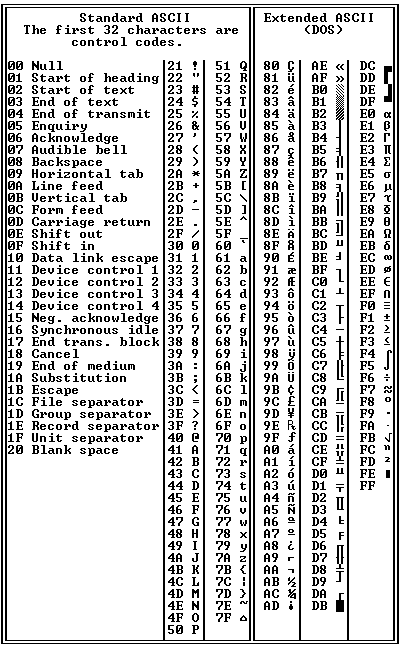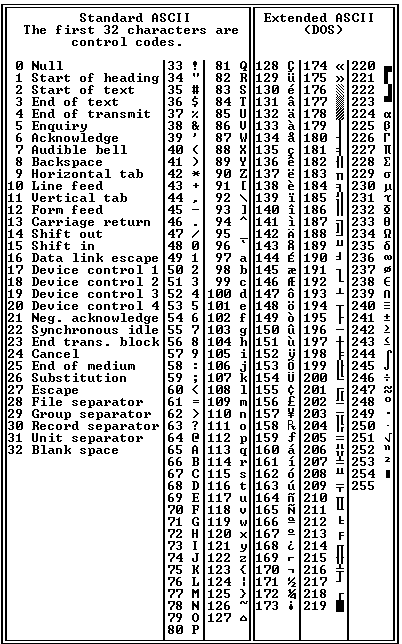hex chart

Also found in: Dictionary, Thesaurus, Medical, Financial.

hex chart

The following charts are ASCII code in hexadecimal (00-FF) and in decimal (0-255). The first 128 characters (00-7F) are standard 7-bit ASCII. The next 128 (80-FF) are extended ASCII as found in the first IBM PC. To view charts with ASCII first, see ASCII chart. See ASCII, hex and hex editor.

ASCII Code (Hexadecimal)The ASCII characters are numbered from 00 to FF (0 to 255). To understand hexadecimal numbering, see hex.

ASCII Code (Decimal)The ASCII characters are numbered from 0 to 255 in decimal. Note that 0 to 9 are the same in both numbering systems.
Site: Follow: Share:
Open / Close